Topics: AI | Data Science | Data Visualization | Deep Learning | Machine Learning | NLP | Python | R | Statistics

KDnuggets Home » News » 2017 » Feb » Tutorials, Overviews » The Gentlest Introduction to Tensorflow – Part 4 ( 17:n08 )

# The Gentlest Introduction to Tensorflow – Part 4

http likes 59

This post is the fourth entry in a series dedicated to introducing newcomers to TensorFlow in the gentlest possible manner, and focuses on logistic regression for classifying the digits of 0-9.

By Soon Hin Khor, Co-organizer for Tokyo Tensorflow Meetup.

Editor's note: You may want to check out part 1, part 2, and part 3 of this tutorial before proceeding.

### Logistic Regression Overview

We have learnt how to use Tensorflow (TF) to perform linear regression to predict an outcome of scalar value, e.g., house prices, given a set of features, e.g., house size.

However, there are times when we want to classify things rather than predict a value, e.g., given an image of a digit we can to classify it as either 0, 1, 2, …, 9, or given a song we want to classify it as pop, rock, rap, etc. Each of the classification in the set [0, 1, 2, …, 9], or [pop, rock, rap, etc.], is known as a class, which in the computer world we represent using a number, e.g., pop = 0, rock = 1, etc. To perform classification, we can employ logistic regression using TF.

In this article, we will use logistic regression to classify the image of a digit, as belonging to classes 0, 1, 2, …, or, 9.

### Logistic Regression Details

The good news is a lot of concepts in linear regression still applies in logistic regression. We can reuse the formula y = W.x + b, but with some tweaks. Let’s look at this formula side-by-side for linear and logistic regression: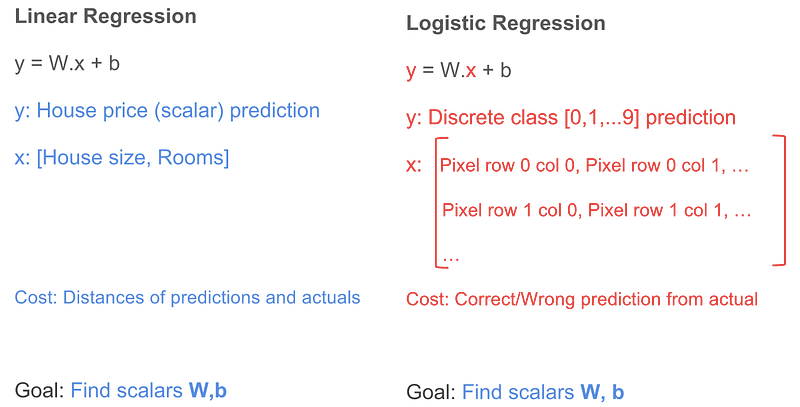Differences & similarities between linear & logistic regression.

Differences:

• Outcome (y): For linear regression, this is a scalar value, e.g., \$50K, \$23.98K, etc. For logistic regression, this is an integer that refers to a class of e.g., 0, 1, 2, .. 9.
• Features (x): For linear regression, each feature is represented as an element in a column vector. For logistic regression involving a 2-D image, this is a 2-dimensional vector, with each element representing a pixel of the image; each pixel has a value of 0–255 representing a grayscale where 0 = black, and 255 = white, and other values some shade of grey.
• Cost function (cost): For linear regression, this is some function calculating the aggregated difference between each prediction and its expected outcome. For logistic regression, this is some function calculating the aggregation of whether each prediction is right or wrong.

Similarity:

• Training: The training goals of both linear and logistic regression are to learn the weights (W) and biases (b) values
• Outcome: The intention of both linear and logistic regression is to predict/classify the outcome (y) with the learned W, and b

### Reconcile Logistic and Linear Regression

To make logistic regression work with y = W.b + x, we need to make some changes to reconcile the differences stated above.

Feature transformation, x

We can convert the 2-dimensional image features in our logistic regression example (assuming it has X rows, Y columns) into a 1-dimensional one (as required in linear regression) by appending each row of pixels one after another to the end of the first row of pixels as shown below.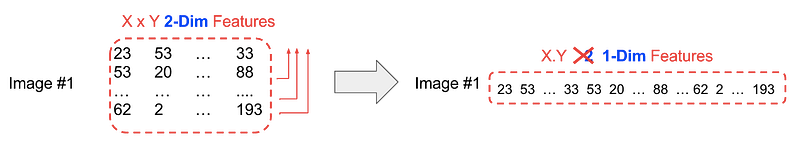Transforming image features to suit logistic regression formula.

Predicted Outcome Transformation, y

For logistic regression, we cannot leave y (predicted outcome) as a scalar since the prediction may end up being 2.3, or 11, which is NOT in the possible classes of [0, 1, …, 9].

To overcome this, the prediction y should be transformed into a single column vector (shown below as row vector to conserve space) where each element represents the score of what the logistic regression model thinks is likely a particular class. In the example below, class ‘1’ is the prediction since it has the highest score.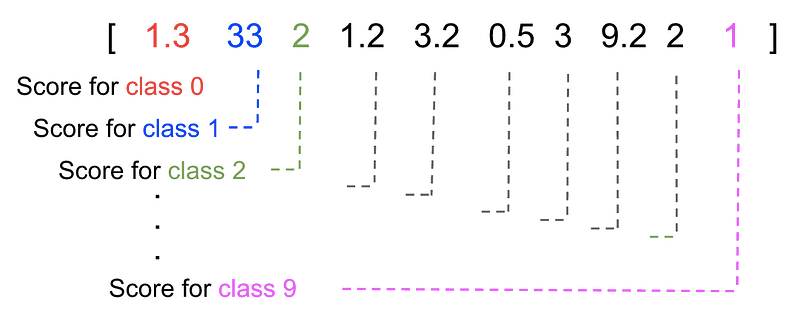Scores for each class and the class with highest score becomes the prediction.

To derive this vector of scores, for a given image, each pixel on it will contribute a set of scores (one for each class) indicating the likelihood it thinks the image is in a particular class, based ONLY on its own greyscale value. The sum of all the scores from every pixel for each class becomes the prediction vector.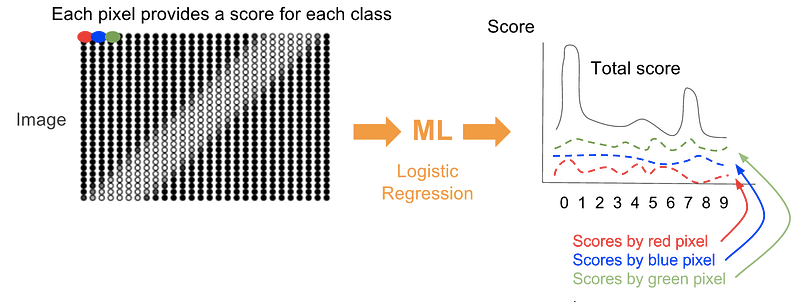Each pixel provides a vector of scores; one score per class, which is becomes the prediction vector. The sum of all prediction vectors becomes the final prediction.

Cost Function Transformation

We cannot use as cost function, any function that involves numerical distance between predicted and actual outcomes. Such a cost function, for an image of ‘1’, will penalize a prediction of ‘7’, more heavily (7–1=6) than a prediction of ‘2’ (2–1=1), although both are equally wrong.

The cost function we are going to use, cross entropy (H) involves multiple steps:

1. Convert actual image class vector (y’) into a one-hot vector, which is a probability distribution
2. Convert prediction class vector (y) into a probability distribution
3. Use cross entropy function to calculate cost, which is the difference between 2 probability distribution function

Step 1. One-hot Vectors

Since we already transformed prediction (y) in to a vector of scores, we should also transform the actual image class (y’) into a vector as well; each element in the column vector represents a class with every element being ‘0’ except the element corresponding to the actual class being ‘1’. This is known as a one-hot vector. Below we show the one-hot vector for each class from 0 to 9.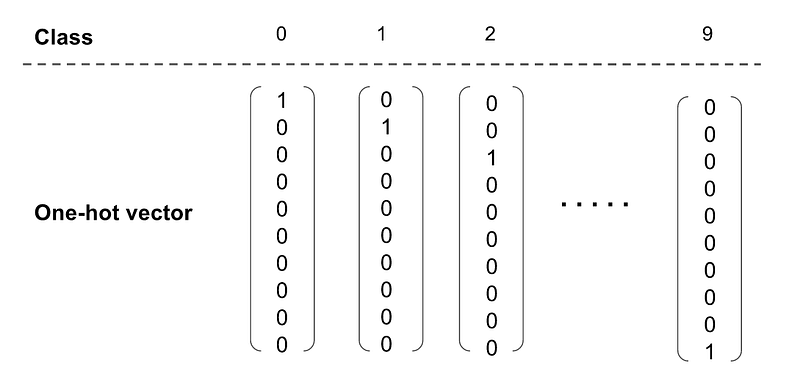Image class and their one-hot vector representations.

Assuming the actual (y’) image being 1, thus having a one-hot vector of [0, 1, 0, 0, 0, 0, 0, 0, 0, 0], and the prediction vector (y) of [1.3, 33, 2, 1.2, 3.2, 0.5, 3, 9.2, 1], plotting them for comparison becomes: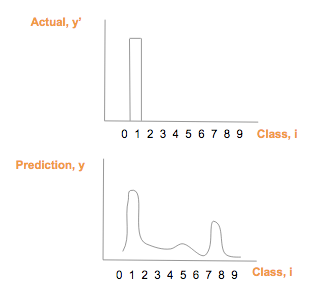Actual image one-hot vector (top) with prediction probability of classes (bottom).

Step 2. Probability Distribution with softmax

To mathematically compare similarity of two ‘graphs’, cross-entropy is a great way (and here is a fantastic albeit long explanation for those with a stomach for details).
To utilize cross entropy however, we need to convert both the actual outcome vector (y’) and the prediction outcome vector (y) values into a ‘probability distribution’, and by ‘probability distribution’ we mean:

• The probability/score of each class has to be between 0 to 1
• The sum of all the probabilities/score for all classes has to be 1

The actual outcome vector (y’) being one-hot vectors already satisfy these constraints.
For prediction outcome vector (y), we can transform it into a probability distribution using softmax: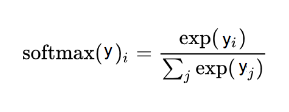softmax equation, where i is the class of 0, 1, 2, …, 9.

This is simply a 2-step process (see S1, S2 below), where each element in the prediction score vector (y), is exp’ed, and divided by the sum of the exp’ed total.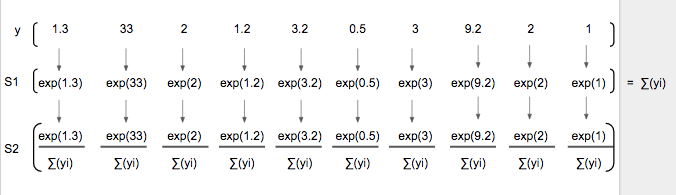Note that softmax(y) graph is similar in shape to the prediction (y) graph but merely with larger max and smaller min values.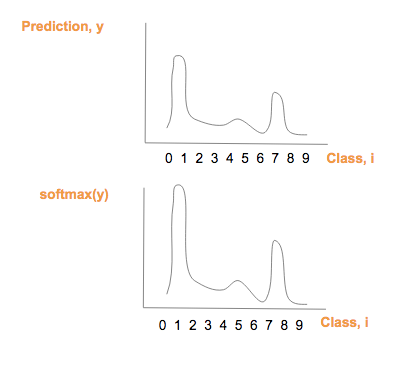Prediction (y) graph before (top) and after applying softmax (below).

Step 3. Cross Entropy

We can now apply cross-entropy (H) between the predicted vector score probability distribution (y’) and the actual vector score probability distribution (y).

The cross entropy formula is: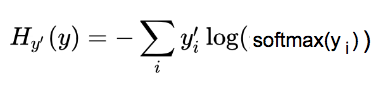Use cross entropy (H) as a cost function that we want to minimize.

To quickly understand this complex formula, we break it down into 3 parts (see below). Note that as notation in this article, we use y_i to represent “y with i subscript” in the formula H:Consider the cross entropy (H) formula as 3 parts: red, blue, red, green.

• Blue: Actual outcome vector, y_i’
• Red: -log of the probability distribution of prediction class vector, (softmax(y_i)), explained previously
• Green: Sum of multiplication of blue and red components for each image class i, where i = 0, 1, 2, …, 9

The illustrations below should simplify understanding further.

The blue plot is just the one-hot vector of actual image class (y’), see One-hot Vector section: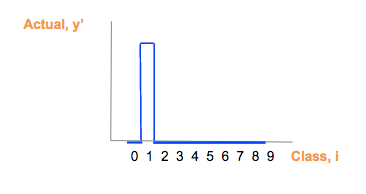The red plot is derived from transformations of each prediction vector element, y, to softmax(y), to -log(softmax(y):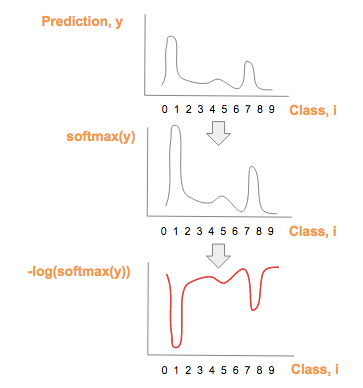Red plot derived from a series of transformation of prediction class vector (y).

If you wish to fully understand why -log(softmax(y)) inverses softmax(y), the second transformation, please check out the video or slides.

The cross entropy (H), the green part (see below) is the multiplication of blue and red values for each class, and then summing them up as illustrated: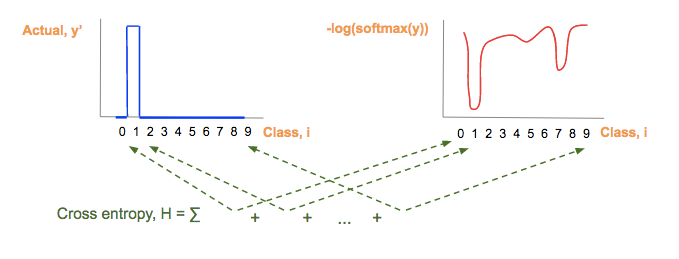Cross entropy (H) is the sum of the multiplication of the blue and red values for each image class.

Since the blue plot is a one-hot vector, it has only a single element of 1, which is for the correct image class, all other multiplications in the cross entropy (H) is 0, and H simplifies to:

`Cross Entropy (H) = -log(softmax(y_i))`

Where:
- y_i: Predicted score/probability for correct image class

### Putting Everything Together

With the 3 proposed transformations, we can now apply the same techniques we used for linear regression, for logistic regression. The code snippets below shows a side-by-side comparison between the linear regression code from Part 3, (available here), and the changes required to make the code work for logistic regression.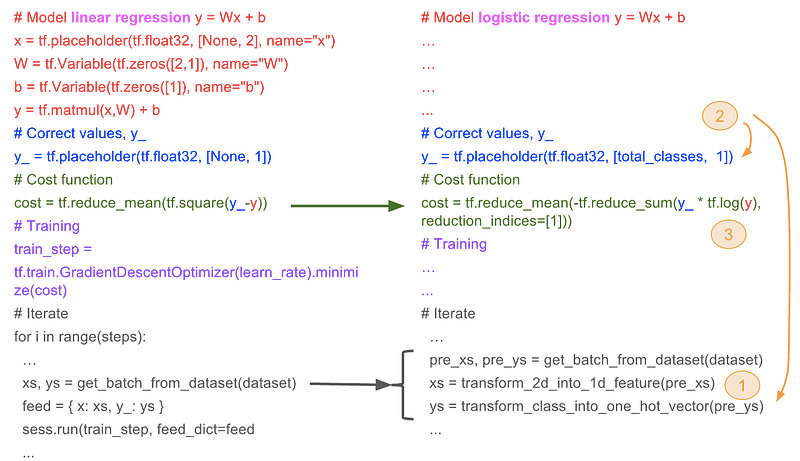Using linear regression techniques for logistic regression. ‘total_class’ is the number of classification classes, e.g., for digits, total_class = 10.

1. Feature (x) transformation to 1-dimensional feature
2. Predicted outcome (y_), and actual outcome (y) transformation to one-hot vectors
3. Cost function transformation from squared error to cross entropy.

The changes can be best summarized in the cheatsheet below: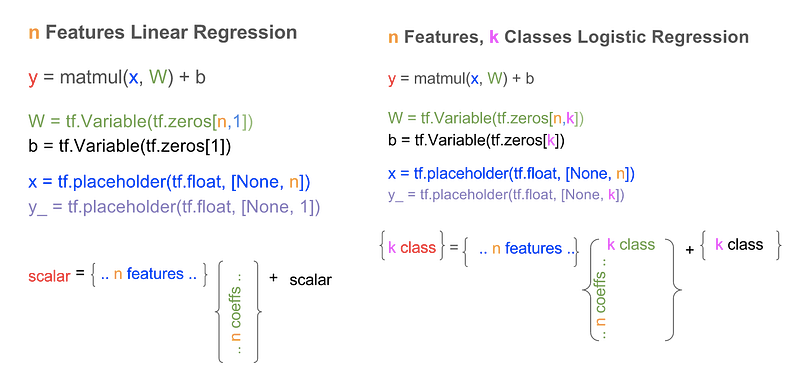Visualizing Linear Regression and Logistic Regression formula/code side-by-side.

### Wrapping Up

Linear regression is useful to predict outcome based on some given features, while logistic regression is useful to help classify an input given the the input’s features.

We show how we can adapt linear regression’s y = W.x + b to work for logistic regression by merely transforming (1) feature vector, x, (2) prediction/outcome vector, y/y’, and (3) cost function, H.

Armed with the knowledge of one-hot vectors, softmax, and cross-entropy, you are now ready to tackle Google’s so-called “beginner’s” tutorial on image classification, which is the goal of this tutorial series.

### Resources

• Google’s code on image classification for beginner’s
• The slides on slideshare

Bio: Soon Hin Khor, Ph.D is using tech to make the world more caring, and responsible. Contributor of ruby-tensorflow. Co-organizer for Tokyo Tensorflow meetup.

Original. Reposted with permission.

Related:

Top Stories Past 30 Days
Most Popular
Most SharedGet KDnuggets, a leading newsletter on AI, Data Science, and Machine Learning